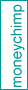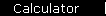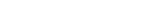# DCF: Discounted Cash Flows Calculator

This calculator finds the fair value of a stock investment the theoretically correct way, as the present value of future earnings. You can find company earnings via the box below.

 Earnings
Earnings per share (last 12 months): \$
 Growth Assumptions
Earnings are expected to grow at a rate of % annually
for the next years,
before leveling off to an annual growth rate of % thereafter.
 Discount Rate
Return available on an appropriate market benchmark investment (like the S&P 500):   %

 Results
Stock Value per share: \$
Show details...

(The "details" screen lets you alter an individual year's earnings if you wish. Its answers can be a few pennies off due to rounding.)

 Find EPS   Ticker:

 Glossary:

Give yourself a "margin of safety" by being conservative in your earnings assumptions. In particular, it's safest to set the long-term earnings growth to zero: if a company actually had constant positive growth forever it would become infinitely big.

(If that didn't convince you, you can also see for yourself how some stock analysts come up with their lofty price targets: keep the discount rate at eleven percent, and then see what happens to the theoretical stock value as you adjust the long-term growth rate from 10% to 10.9% to 10.99%... You'll find that you can force the math to give you any answer you want. So play it safe: if you want a meaningful answer that you can really understand, always assume that long-term growth is zero.)

So far we've gone over valuation theory and used one calculator (and if you want you can also see the formula that makes this all work) ... and that's about all there is to it.

What follows is a series of shortcuts - simple ratios people use to estimate valuation quickly - and some variations.

home  |  article  |  glossary  |  calculator  |  about us  |  booksValuation IntroA Little TheoryDCF CalculatorP/E RatioP/S RatioPEG RatioGraham FormulaDividend DiscountBuffett Formula (?)CAPM CalculatorBooks & LinksValuation FormulaStock Market PredictionsMeasuring Investment ReturnsStock Market CAGR